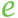# E

 Block Group: Constants Icon:The E block is a Number block. When the E block is created, the E block holds the mathematical constant e.

For information on using dataflow blocks, see Dataflow.

## Input/Output Property

The following property of the E block can take input and give output.

• value (number)

value sets and returns the number held by this E block. When an E block is created, the value property holds the mathematical constant e.

## Example

The following image demonstrates an example of the E block.